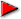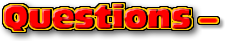Chapterspage 1This text is meant to accompany class discussions. It is not everything there is to know about uniform circular motion. It is meant as a  prep for class. More detailed notes and examples are given in the class notes, presentations, and demonstrations (click here.)Click for the questions that go with this reading
Displacement and Constant Velocity

The assumption is that you have read through the pre-reading, click here.

To move at a constant velocity means that the speed remains unchanged and the acceleration is also zero. It is constantly zero. This motion is called "constant velocity."

The variable Δx is the displacement. Displacement is the change in position. Therefore the displacement is the final position minus the initial position. Mathematically it is written as Δx = x - xO where

 Δx = the displacement (change in position) x = final position xO = initial position

Usually for problem solving the initial position, xO, is equal to zero. This will make some of our problem solving easier.

There four types of velocities to be considered:

 Δv = the change in velocity vO = initial velocity v = final velocity vAVG = average velocity

Similar to displacement, Δv = v - vO. Any time the change in a quantity is mentioned it is always measured as the final amount minus the initial amount. The final amount occurs later in time.

Usually when people think of velocity, they think of moving fast or slow. But velocity can also be though of as how quickly you change position. Velocity times time is the displacement –or change in position.Here is how to visualize a simple constant velocity problem.

 Example A dog is playing "fetch" by chasing a ball a returning it to his boy. The dog is exactly 5 meters away from the boy when the boy throws the ball past the dog. The dog walks after the ball at a constant velocity of 2 m/s for 3 seconds. How far away was the ball when it landed?by Tony Wayne ...(If you are a teacher, please feel free to use these resources in your teaching.)